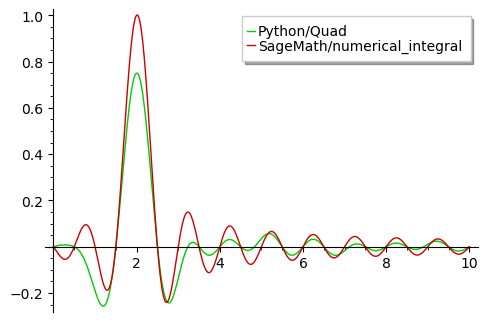2023-09-21 10:38:46 +0100 received badge ● Notable Question (source) 2022-12-29 18:29:28 +0100 received badge ● Popular Question (source) 2022-12-20 10:47:53 +0100 received badge ● Popular Question (source) 2021-08-22 16:08:46 +0100 commented answer Difference between numerical_integral and scipy.integrate.quad I'm using Sage 9.3 2021-08-22 13:34:55 +0100 marked best answer Difference between numerical_integral and scipy.integrate.quad I plot an integral with quad() function in a Python file. I wanted to plot the same result with SageMath but the values returned are different and I really don't understand why the two graphics are not the same ... Here is my Sage code : import scipy.integrate as sp_int int1(a, j, k, L) = piecewise([[[0, 1/4], 1 * cos(pi * (j + 1) * a/L) * cos(pi * (k + 1) * a/L)], [(1/4, 3/4), 2 * cos(pi * (j + 1) * a/L) * cos(pi * (k + 1) * a/L)], [[3/4, 1], 1 * cos(pi * (j + 1) * a/L) * cos(pi * (k + 1) * a/L)]]) def int2(a, j, k, L): if a < 1 / 4 or a > 3 / 4: return cos(pi * (j + 1) * a/L) * cos(pi * (k + 1) * a/L) else: return 2* cos(pi * (j + 1) * a/L) * cos(pi * (k + 1) * a/L) def integral1(j): k = 2 L = 0.5 return numerical_integral(int1, 0, 1, max_points=10, params=[j, k, L]) def integral2(j): k = 2 L = 0.5 return sp_int.quad(int2, 0, 1, args=(j, k, L)) i1 = plot(integral1, 0, 10, rgbcolor=(0.8,0, 0), legend_label='SageMath/numerical_integral') i2 = plot(integral2, 0, 10, rgbcolor=(0, 0.8, 0), legend_label='Python/Quad') show(i2 + i1)2021-08-22 13:34:55 +0100 received badge ● Scholar (source) 2021-08-22 08:55:33 +0100 commented answer Difference between numerical_integral and scipy.integrate.quad What a great answer ! Deep but clear explanation. I learned a lot from reading you. Thanks. 2021-08-22 08:38:52 +0100 received badge ● Supporter (source) 2021-08-21 16:36:11 +0100 commented question Difference between numerical_integral and scipy.integrate.quad Thanks for your comments. Frédéric, certes ... : How to piecewise on 'a' ? Tolga : I will try 2021-08-21 16:35:45 +0100 commented question Difference between numerical_integral and scipy.integrate.quad Thanks for your comments. - Frédéric, certes ... : How to piecewise on 'a' ? - Tolga : I will try 2021-08-21 16:35:17 +0100 commented question Difference between numerical_integral and scipy.integrate.quad Thanks for your comments - Frédéric, certes ... : How to piecewise on 'a' ? - Tolga : I will try 2021-08-21 16:24:09 +0100 commented question Difference between numerical_integral and scipy.integrate.quad Frédéric : How to piecewise on 'a' ? Tolga : I will try 2021-08-21 16:23:53 +0100 commented question Difference between numerical_integral and scipy.integrate.quad Frédéric : How to piecewise on 'a' ? Tolga : I will try 2021-08-21 16:17:22 +0100 edited question Difference between numerical_integral and scipy.integrate.quad Difference between numerical_integral and spicy.integrate.quad I plot an integral with quad() function in a Python file. 2021-08-21 12:55:13 +0100 received badge ● Editor (source) 2021-08-21 12:55:13 +0100 edited question Difference between numerical_integral and scipy.integrate.quad Difference between numerical_integral and spicy.integrate.quad I plot an integral with quad() function in a Python file. 2021-08-21 12:38:32 +0100 asked a question Difference between numerical_integral and scipy.integrate.quad Difference between numerical_integral and spicy.integrate.quad I plot an integral with quad() function in a Python file. 2021-08-08 12:31:56 +0100 commented answer How to concatenate 2 vectors in a third ... Smart ! Danke I will create & use a simple function : concatene_vectors(a,b): return vector(chain(a,b)) 2021-08-08 12:30:11 +0100 commented answer How to concatenate 2 vectors in a third ... Smart ! I will create & use a simple function : concatene_vectors(a,b): return vector(chain(a,b)) 2021-08-08 12:29:37 +0100 commented answer How to concatenate 2 vectors in a third ... Smart ! I will create & use a simple function : concatene_vectors(a,b): return vector(chain(a,b)) 2021-08-08 12:29:25 +0100 commented answer How to concatenate 2 vectors in a third ... Smart ! I will create & use a simple function : concatene_vectors(a,b): return vector(chain(a,b)) 2021-08-08 12:28:41 +0100 commented answer How to concatenate 2 vectors in a third ... Smart ! I will create & use a simple function : concatene_vectors(a,b): return vector(chain(a,b)) 2021-08-08 11:01:44 +0100 asked a question How to concatenate 2 vectors in a third ... How to concatenate 2 vectors in a third ... Hi. I can't find in the documentation an elegant solution for this quite sim 2021-08-02 20:48:35 +0100 commented answer No png when using a file It works perfectly ! Thanks a lot 2021-08-02 20:46:28 +0100 answered a question No png when using a file It works perfectly ! Thanks a lot 2021-08-02 14:53:23 +0100 received badge ● Student (source) 2021-08-02 14:41:28 +0100 asked a question No png when using a file No png when using a file Hi, I'm using SageMath version 9.3, Release Date: 2021-05-09 If I write in my terminal : print